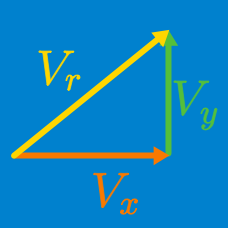Classical Mechanics

# Scalar multiplication of velocity vectors

A velocity vector $\vec{v}$ of magnitude $7.0 \text{ m/s}$ is directed south. What is the direction of the vector $-5.0 \vec{v}?$

The motion of a creature of mass $2 \text{ kg}$ moving on a two dimensional plane can be described by the following equations: \begin{aligned} v_x(t)&=5t + 2 \text{ (m/s)} \\ v_y(t)&=2t - 2 \text{ (m/s)}, \end{aligned} where $v_x(t)$ and $v_y(t)$ denote the velocities in the $x$ and $y$ directions, respectively. Find the creature's momentum vector at $t = 3 \text{ s}$ in unit-vector notation.

The motion of a creature of mass $6 \text{ kg}$ on a two dimensional plane can be described by the following equations: \begin{aligned} a_x(t)&= 4 \text{ m/s}^2 \\ a_y(t)&= -1 \text{ m/s}^2, \end{aligned} where $a_x(t)$ and $a_y(t)$ denote the accelerations in the positive directions of the $x$-axis and $y$-axis, respectively. If the creature is initially at rest, what is the creature's velocity vector at $t = 2 \text{ s}$ in unit-vector notation?

A ship sails on the $xy$-plane in a direction that makes a $60 ^{\circ}$ angle with the positive direction of the $x$-axis, at a speed of $27 \text{ m/s}.$ Find the velocity vector of this ship in unit-vector notation.

The motion of a creature of mass $6 \text{ kg}$ on a two dimensional plane can be described by the following equations: \begin{aligned} v_x(t)&=t^2 + 4\text{ (m/s)} \\ v_y(t)&=3t - 1 \text{ (m/s)}, \end{aligned} where $v_x(t)$ and $v_y(t)$ denote the velocities in the $x$ and $y$ directions, respectively. Find the creature's momentum vector at $t = 2 \text{ s}$ in unit-vector notation.

×